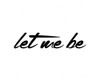Let Me Be

\$32.70

Let Me Be

\$147.33

Let Me Be

\$45.99

Let Me Be

\$49.23

Let Me Be

\$16.07

Let Me Be

\$38.00

Let Me Be

\$25.08

Let Me Be

\$16.45

Let Me Be

\$15.43

Let Me Be

\$17.23

Let Me Be

\$89.78